Students can download Maths Chapter 6 Trigonometry Ex 6.3 Questions and Answers, Notes, Samacheer Kalvi 9th Maths Guide Pdf helps you to revise the complete Tamilnadu State Board New Syllabus, helps students complete homework assignments and to score high marks in board exams.

## Tamilnadu Samacheer Kalvi 9th Maths Solutions Chapter 6 Trigonometry Ex 6.3

Question 1.
Find the value of the following: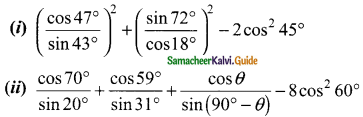(iii) tan 15° tan 30° tan 45° tan 60° tan 75°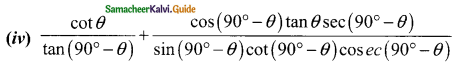Solution:
(i) cos 45° = $$\frac{1}{√2}$$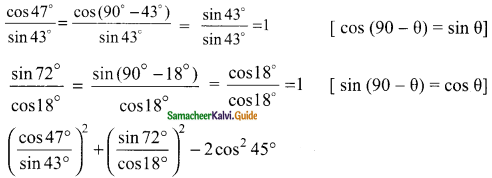= 1² + 1² – 2($$\frac{1}{√2}$$)²
= 1 + 1 – 2($$\frac{1}{2}$$)
= 2 – 1
= 1(ii) cos 60° = $$\frac{1}{2}$$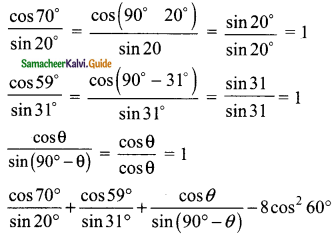= 1 + 1 + 1 – 8($$\frac{1}{2}$$)²
= 3 – 8 × $$\frac{1}{4}$$
= 3 – 2
= 1(iii) tan 30° = $$\frac{1}{√3}$$, tan 45° = 1, tan 60° = √3
tan 15° . tan 30°. tan 45° . tan 60°. tan 75° = tan 15° . $$\frac{1}{√3}$$ . 1 . √3 tan 75°
= tan 15° × tan 75° × $$\frac{1}{√3}$$ × 1 × √3
= tan(90° – 75°) × $$\frac{1}{cot 75°}$$ × 1 [tan 90° – θ = cot θ]
= cot 75° × $$\frac{1}{cot 75°}$$ × 1
= 1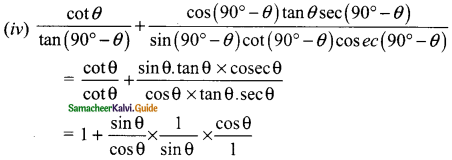= 1 + 1
= 2